• ## 图像预处理

千次阅读 2019-12-18 13:07:11
文章目录为什么需要图像预处理图像预处理流程一，灰度化（1）分量法（2）最大值法（3）平均值法（4）加权平均法二，几何变换（1）opencv来进行图像的空间变换（2）双线性插值算法实现缩放三，图像增强（1）频率域...
文章目录为什么需要图像预处理？图像预处理流程一，灰度化（1）分量法（2）最大值法（3）平均值法（4）加权平均法二，几何变换（1）opencv来进行图像的空间变换（2）双线性插值算法实现缩放三，图像增强（1）频率域法（2）空间域法
为什么需要图像预处理？
图像预处理的主要目的是消除图像中无关的信息，恢复有用的真实信息，增强有关信息的可检测性和最大限度地简化数据，从而改进特征抽取、图像分割、匹配和识别的可靠性。
图像预处理流程
一般的图像预处理步骤为：灰度化 ——>几何变换——>图像增强
一，灰度化
灰度化，在RGB模型中，如果R=G=B时，则彩色表示一种灰度颜色，其中R=G=B的值叫灰度值，因此，灰度图像每个像素只需一个字节存放灰度值（又称强度值、亮度值），灰度范围为0-255。一般有分量法，最大值法，平均值法，加权平均法四种方法对彩色图像进行灰度化。
（1）分量法
三个灰度图像的灰度值是彩色图像中三分量中任意一个分量的亮度值，可以是R分量作为灰度值，也可以是G分量和B分量
f1(i,j)=R(i,j)f2(i,j)=G(i,j)f3(i,j)=B(i,j)
from PIL import Image
import numpy as np

def image2array(image):
"""
:param image: 图片
:return: 图片的数组
"""
image = np.array(image)
return image

def array2image(arrimg):
"""
:param arrimg: 图片的数组
:return: 图片
"""
image = Image.fromarray(arrimg.astype('uint8')).convert('RGB')
return image

def convert2gray(img):
"""
:param img: 数组形式的图片
:return: 分量法灰度化后的图片数组
"""
if len(img.shape) > 2:
r, g, b = img[:, :, 0], img[:, :, 1], img[:, :, 2]
gray = r  # 可以是r,也可以是g，b
return gray
else:
return img

if __name__ == '__main__':
image = Image.open("a.jpg")
image = image2array(image)
image = convert2gray(image)
image = array2image(image)
image.show()

（2）最大值法
f(i,j)=max(R(i,j),G(i,j),B(i,j))
def convert2gray(img):
"""
:param img: 图片的数组
:return: 最大值法灰度化后的图片数组
"""
grayimg = np.zeros(shape=(img.shape,img.shape))
if len(img.shape) > 2:
for i in range(img.shape):
for j in range(img.shape):
grayimg[i,j] = max(img[i,j], img[i,j], img[i,j])
return grayimg
else:
return img

（3）平均值法
f(i,j)=(R(i,j)+G(i,j)+B(i,j)) /3
def convert2gray(img):
"""
:param img: 图片的数组
:return: 最大值法灰度化后的图片数组
"""
"""
:param img: 图片的数组
:return: 平均法灰度化后的图片数组
"""
grayimg = np.zeros(shape=(img.shape, img.shape))
if len(img.shape) > 2:
for i in range(img.shape):
for j in range(img.shape):
grayimg[i, j] = (int(img[i, j])+int(img[i, j])+int(img[i, j]))/3
return grayimg
else:
return img

（4）加权平均法
f(i,j)=0.2989R(i,j)+0.5870G(i,j)+0.1140B(i,j)
def convert2gray(img):
"""
:param img: 数组形式的图片
:return: 加权平均法灰度化后的图片数组
"""
if len(img.shape) > 2:
r, g, b = img[:, :, 0], img[:, :, 1], img[:, :, 2]
gray = 0.2989 * r + 0.5870 * g + 0.1140 * b
return gray
else:
return img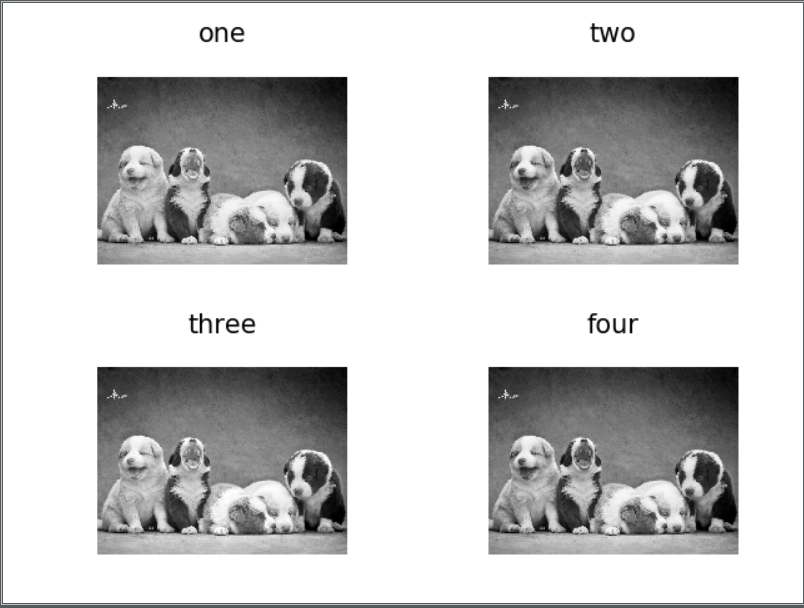二，几何变换
图像几何变换又称为图像空间变换，通过平移、转置、镜像、旋转、缩放等几何变换对采集的图像进行处理，用于改正图像采集系统的系统误差和仪器位置（成像角度、透视关系乃至镜头自身原因）的随机误差。此外，还需要使用灰度插值算法，因为按照这种变换关系进行计算，输出图像的像素可能被映射到输入图像的非整数坐标上。通常采用的方法有最近邻插值、双线性插值和双三次插值
（1）opencv来进行图像的空间变换
#!/usr/bin/python3
# __*__ coding: utf-8 __*__
import cv2
import numpy as np
from matplotlib import pyplot as plt
from pylab import mpl
mpl.rcParams['font.sans-serif'] = ['FangSong'] # 指定默认字体
mpl.rcParams['axes.unicode_minus'] = False # 解决保存图像是负号'-'显示为方块的问题

"""
尺度变换
尺度变换(缩放，拉伸)是调整图像大小，使其变大或变小。
但实际操作时要比想象的复杂一些，因为调整大小带来了像素如何插值（放大）或合并（减少）的问题。
cv2.resize(src,dsize,fx=0,fy=0,interpolation=cv2.INTER_LINEAR)
cv2.INTER_LINEAR	双线性插值法
cv2.INTER_NEAREST	最近邻插值
cv2.INTER_AREA	像素区域重采样(默认)
cv2.INTER_CUBIC	双三次插值
cv2.INTER_LANCZ0S4	插值（超过8×8个邻域）
"""
# 绝对尺寸
height,width = img1.shape[:2]
res = cv2.resize(img1,(2*width,2*height),interpolation=cv2.INTER_CUBIC)
# 相对尺寸
res1 = cv2.resize(img1,None,fx=0.3,fy=0.3)

"""
平移
cv2.warpAffine(src, M, dsize)
src：输入图像
M：移动矩阵
dsize：输出图像大小
"""
tx = 500
ty = 100
M = np.float32([[1,0,tx],[0,1,ty]])
dst = cv2.warpAffine(img1,M,(img1.shape+tx,img1.shape+ty))

tx = 500
ty = 200
M = np.float32([[1,0,tx],[0,1,ty]])
dst1 = cv2.warpAffine(img1,M,(1300,1000))

"""
旋转变换
cv2.getRotationMatrix2D(center, angle, scale)
cv2.warpAffine(src, M, dsize[, dst[, flags[, borderMode[, borderValue]]]]) → dst
center–表示旋转的中心点
angle–表示旋转的角度degrees
scale–图像缩放因子

src – 输入的图像
M – 2 X 3 的变换矩阵.
dsize – 输出的图像的size大小
dst – 输出的图像
flags – 输出图像的插值方法(参考尺度变换)
borderMode – 图像边界的处理方式
"""
rows, cols = img1.shape[:2]
# 这里(cols / 2, rows / 2)为旋转中心，60旋转角度，第三个为旋转后的缩放因子
# 可以通过设置旋转中心，缩放因子，以及窗口大小来防止旋转后超出边界的问题
M = cv2.getRotationMatrix2D((cols / 2, rows / 2), 60, 1)
dst2 = cv2.warpAffine(img1, M, (cols, rows))

"""
翻转
cv2.flip(src, flipCode[, dst]) → dst1
src – 输入的图像
dst – 输出的图像
flipCode – 翻转模式：
flipCode==0垂直翻转（沿X轴翻转）
flipCode>0水平翻转（沿Y轴翻转）
flipCode<0水平垂直翻转（先沿X轴翻转，再沿Y轴翻转，等价于旋转180°）
"""
# 水平翻转
dst3 = cv2.flip(img1, 1)
# 垂直翻转
dst4 = cv2.flip(img1, 0)
# 水平垂直翻转
dst5 = cv2.flip(img1, -1)

"""
仿射变换
仿射变换具体到图像中的应用，主要是对图像的缩放scale，
旋转rotate，剪切shear，翻转flip和平移translate的组合
"""
pts1 = np.float32([[500,500],[2000,500],[500,2000]])
pts2 = np.float32([[100,1000],[2000,500],[1000,2500]])
M = cv2.getAffineTransform(pts1,pts2)
img2 = cv2.warpAffine(img1,M,(rows,cols))

"""
透视变换
"""
pts1 = np.float32([[200,200],[200,3000],[4800,200],[4800,3000]])
pts2 = np.float32([[200,200],[200,3000],[4800,600],[4800,2000]])
M = cv2.getPerspectiveTransform(pts1,pts2)
img3=cv2.warpPerspective(img1,M,(cols,rows),0.6)

# 图像显示
plt.figure()

plt.subplot(431)
plt.title("原始")
plt.imshow(img1)

plt.subplot(432)
plt.title("绝对扩大")
plt.imshow(res)

plt.subplot(433)
plt.title("相对缩小多少倍")
plt.imshow(res1)

plt.subplot(434)
plt.title("平移(右移500，下移100)")
plt.imshow(dst)

plt.subplot(435)
plt.title("平移(右移500，下移200)")
plt.imshow(dst1)

plt.subplot(436)
plt.title("旋转60度")
plt.imshow(dst2)

plt.subplot(437)
plt.title("水平翻转")
plt.imshow(dst3)

plt.subplot(438)
plt.title("垂直翻转")
plt.imshow(dst4)

plt.subplot(439)
plt.title("水平垂直翻转")
plt.imshow(dst5)

plt.subplot(4,3,10)
plt.title("仿射变换")
plt.imshow(img2)

plt.subplot(4,3,11)
plt.title("透视变换")
plt.imshow(img3)
plt.show()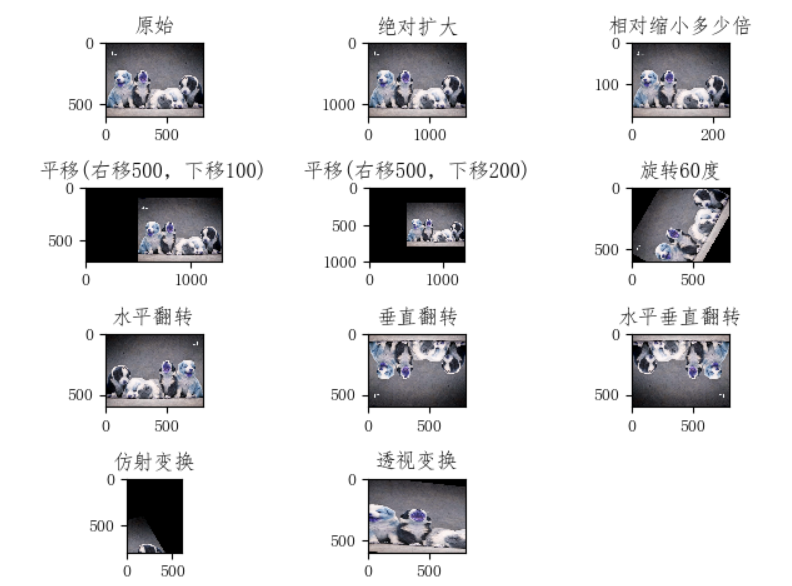（2）双线性插值算法实现缩放
双线性插值的特点：
1.计算过程中充分的考虑到了各邻点的特征，具有灰度平滑过渡的特点；
2.一般情况下可以得到满意的结果；
3.具有低通滤波的特性，使图像轮廓变的模糊；
4.平滑作用会使图像细节退化，尤其是在放的的时候；
5.不连续性会产生不希望的结果。
#!/usr/bin/python3
# __*__ coding: utf-8 __*__
'''
双线性插值图像缩放算法
'''
import numpy as np
import cv2 as cv
import math

def bi_linear(src, dst, target_size):
th, tw = target_size, target_size
emptyImage = np.zeros(target_size, np.uint8)
for k in range(3):
for i in range(th):
for j in range(tw):
# 首先找到在原图中对应的点的(X, Y)坐标
corr_x = (i+0.5)/th*pic.shape-0.5
corr_y = (j+0.5)/tw*pic.shape-0.5
point1 = (math.floor(corr_x), math.floor(corr_y))   # 左上角的点
point2 = (point1, point1+1)
point3 = (point1+1, point1)
point4 = (point1+1, point1+1)

fr1 = (point2-corr_y)*pic[point1, point1, k] + (corr_y-point1)*pic[point2, point2, k]
fr2 = (point2-corr_y)*pic[point3, point3, k] + (corr_y-point1)*pic[point4, point4, k]
emptyImage[i, j, k] = (point3-corr_x)*fr1 + (corr_x-point1)*fr2

cv.imwrite(dst, emptyImage)

def main():
src = 'a.jpg'
dst = 'b.png'
target_size = (300, 200, 3)     # 变换后的图像大小

bi_linear(src, dst, target_size)

if __name__ == '__main__':
main()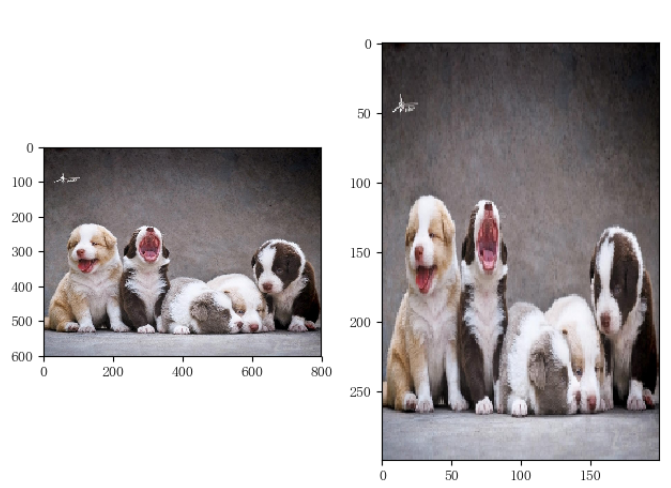三，图像增强
增强图像中的有用信息，它可以是一个失真的过程，其目的是要改善图像的视觉效果，针对给定图像的应用场合，有目的地强调图像的整体或局部特性，将原来不清晰的图像变得清晰或强调某些感兴趣的特征，扩大图像中不同物体特征之间的差别，抑制不感兴趣的特征，使之改善图像质量、丰富信息量，加强图像判读和识别效果，满足某些特殊分析的需要。
图像增强可分成两大类：频率域法和空间域法。
（1）频率域法
频率域法把图像看成一种二维信号，对其进行基于二维傅里叶变换的信号增强。
高频图像是指强度变化很多的图像，亮度水平从一个像素到另一个像素变化很快。低频图像可能是亮度比较均匀或变化很慢的图像。
在频谱中，图像中心处对应了图像中变化较平缓的区域，四周对应了图像边缘、噪音或者变化陡峭的部分。
因此我们可以将代表了图像边缘、噪音或者变化陡峭的高频率成分滤除掉，只留下变化平缓的低频率成分，再由频域变换回时域，这就相当于对图像进行了平滑处理；相反，若滤去低频率成分，只留下高频率成分，就相当于对图像进行了锐化处理
频域增强的原理十分简单，其应用的重点问题在于滤波半径阈值如何选取。
滤波数学表达式：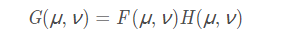其中G(x,y)、 H(u,v)、F(u,v)分别是g(x,y)、h(x,y)、f(x,y)的傅立叶变换。
常用的高通滤波器：
高通滤波器 = 1- 低通滤波器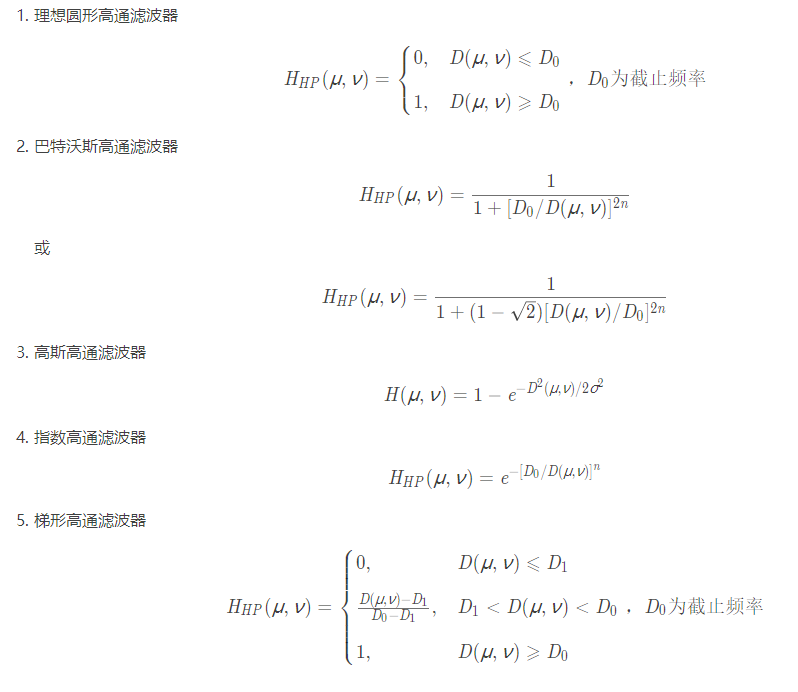# coding = utf-8
import numpy as np
import matplotlib.pyplot as plt
from PIL import Image

def array2image(arrimg):
"""
:param arrimg: 图片的数组
:return: 图片
"""
image = Image.fromarray(arrimg.astype('uint8')).convert('RGB')
return image

def make_transform_matrix(image_arr, d0, ftype='low'):
"""
构建高斯高/低通滤波
:param image_arr: 图像数组
:param d0: 通带半径
:param ftype: 类型
:return: 滤波器
"""
# 构建滤波器
transfor_matrix = np.zeros(image_arr.shape, dtype=np.float32)
w, h = image_arr.shape
for i in range(w):
for j in range(h):
distance = np.sqrt((i - w / 2) ** 2 + (j - h / 2) ** 2)
# Gaussian滤波函数
transfor_matrix[i, j] = np.e ** (-1 * (distance ** 2 / (2 * d0 ** 2)))
if ftype == 'low':
return transfor_matrix
elif ftype == 'high':
return 1 - transfor_matrix

# 图像灰度化
img_arr = np.array(Image.open('a.jpg').convert('L'))

# 将图像从空间域转换到频率域，傅里叶变换
f = np.fft.fft2(img_arr)
fshift = np.fft.fftshift(f)

# 生成低通滤波器
F_filter1 = make_transform_matrix(img_arr, 30, 'low')
# 滤波
result = fshift * F_filter1
# 将图像从频率域转换到空间域，傅里叶反变换
img_d1 = np.abs(np.fft.ifft2(np.fft.ifftshift(result)))

# 可视化
plt.imshow(array2image(img_d1))
plt.show()

（2）空间域法
空间域图像增强技术指在空间域中，通过线性和非线性变换来增强构成图像的像素
增强的方法主要分为点运算算法和邻域去噪算法
点运算算法即灰度级校正、灰度变换（伽马变换、对数增强）和直方图修正等，目的或使图像成像均匀，或扩大图像动态范围，扩展对比度。
邻域增强算法分为图像平滑和锐化两种。平滑一般用于消除图像噪声，但是也容易引起边缘的模糊。常用算法有均值滤波、中值滤波。锐化的目的在于突出物体的边缘轮廓，便于目标识别。锐化常用算法有梯度法（如Roberts梯度法）、算子法（Sobel算子和拉普拉斯算子等）、掩模匹配法、统计差值法等常用算法有梯度法、算子、高通滤波、掩模匹配法、统计差值法等。
（1）点运算算法
from skimage import exposure
import numpy as np
from PIL import Image
from matplotlib import pyplot as plt
from pylab import mpl
mpl.rcParams['font.sans-serif'] = ['FangSong'] # 指定默认字体
mpl.rcParams['axes.unicode_minus'] = False # 解决保存图像是负号'-'显示为方块的问题

def image2array(image):
"""
:param image: 图片
:return: 图片的数组
"""
image = np.array(image)
return image
def array2image(arrimg):
"""
:param arrimg: 图片的数组
:return: 图片
"""
image = Image.fromarray(arrimg.astype('uint8')).convert('RGB')
return image

def image_gamma_transform(pil_im, gamma):
"""
伽马变换
:param pil_im: 图片
:return: 处理后的图片
"""
image_arr = np.array(pil_im)
return array2image(image_arr2)

def image_gamma_transform2(pil_im, gamma):
"""
伽马变换2（源码实现)
:param pil_im: 图片
:return: 处理后的图片
"""
image_arr = np.array(pil_im)
image_arr2 = np.power(image_arr / float(np.max(image_arr)), gamma)
return array2image(image_arr2*float(np.max(image_arr)))

def image_log_transform(pil_im):
"""
图像对数增强
:param pil_im: 图片
:return: 处理后的图片
"""
image_arr = image2array(pil_im)
if len(image_arr.shape) == 3:
for i in range(3):
image_arr[:,:,i] = 255/np.log(255+1)*np.log(1+image_arr[:,:,i])
return array2image(image_arr)
elif len(image_arr.shape) == 2:
# image_arr = 255/np.log(np.max(image_arr)+1)*np.log(1+image_arr)
image_arr = 255/np.log(255+1)*np.log(1+image_arr)
return array2image(image_arr)

def image_histeq(pil_im):
"""
直方图均衡化
:param pil_im: 图片
:return: 处理后的图片
"""
# 计算图像的直方图
image_arr = image2array(pil_im)
imhist, bins = np.histogram(image_arr.flatten(), 256, normed=True)
cdf = imhist.cumsum()   # 累计分布函数
cdf = 255*cdf/cdf[-1]   # 归一化
# 使用累计分布函数的线性插值计算新的像素值
image_arr2 = np.interp(image_arr.flatten(), bins[:-1], cdf)
return array2image(image_arr2.reshape(image_arr.shape))

if __name__ == '__main__':
image = Image.open('a.jpg').convert('L')
image1 = image_gamma_transform(image,2.7)
image2 = image_gamma_transform2(image,0.3)
image3 = image_log_transform(image)
image4 = image_histeq(image)
plt.figure()
plt.subplot(221)
plt.title("伽马变换,gamma值设置为2.7")
plt.imshow(image1)
plt.subplot(222)
plt.title("伽马变换,gamma值设置为0.3")
plt.imshow(image2)
plt.subplot(223)
plt.title("图像对数增强")
plt.imshow(image3)
plt.subplot(224)
plt.title("直方图均衡化")
plt.imshow(image4)
plt.show()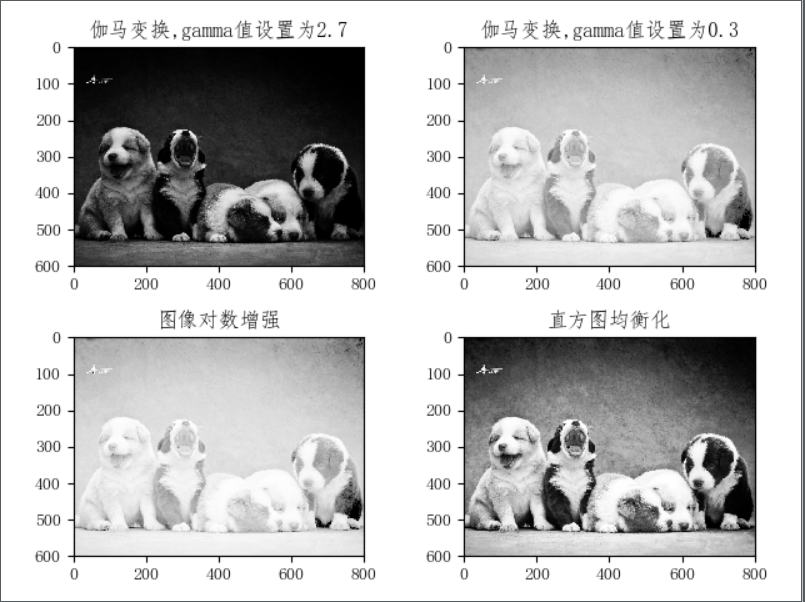（2）领域去噪算法
#!/usr/bin/python3
# __*__ coding: utf-8 __*__
from scipy.ndimage import filters
from scipy.signal import convolve2d
from skimage import exposure
import numpy as np
from PIL import Image
from matplotlib import pyplot as plt
from pylab import mpl
mpl.rcParams['font.sans-serif'] = ['FangSong'] # 指定默认字体
mpl.rcParams['axes.unicode_minus'] = False # 解决保存图像是负号'-'显示为方块的问题
def image2array(image):
"""
:param image: 图片
:return: 图片的数组
"""
image = np.array(image)
return image
def array2image(arrimg):
"""
:param arrimg: 图片的数组
:return: 图片
"""
image = Image.fromarray(arrimg.astype('uint8')).convert('RGB')
return image

def image_mean_filter(pil_im):
"""
均值滤波
:param pil_im: 图片
:return: 处理后的图片
"""
image_arr = image2array(pil_im)
dst_arr = np.zeros_like(image_arr)
# 卷积核-均值算子
mean_operator = np.array([[1, 1, 1],
[1, 1, 1],
[1, 1, 1]]) / 9
if len(image_arr.shape) == 3:
for i in range(3):
dst_arr[:, :, i] = convolve2d(image_arr[:, :, i], mean_operator, mode="same")
elif len(image_arr.shape) == 2:
dst_arr = convolve2d(image_arr, mean_operator, mode="same")

return array2image(dst_arr)

def image_medium_filter(pil_im, sigma=5):
"""
中值滤波
中值平滑只对特别尖锐的信号平滑
:param pil_im: 图片
:return: 处理后的图片
"""
image_arr = image2array(pil_im)
if len(image_arr.shape) == 3:
for i in range(3):
image_arr[:,:,i] = filters.median_filter(image_arr[:,:,i], sigma)
return image_arr
elif len(image_arr.shape) == 2:
image_arr = filters.median_filter(image_arr, sigma)
return array2image(image_arr)

def image_laplace_filter(pil_im,sigma):
'''
拉普拉斯算子增强
sigma越大图像越模糊
INPUT  -> 单张图文件
OUTPUT -> 处理后的图文件
'''
image_arr = np.array(pil_im)
dst_arr = np.zeros(image_arr.shape, dtype=np.uint8)
filter_arr = dst_arr
# 卷积核-拉普拉斯算子
laplace_operator = np.array([[0, -1, 0],
[-1, 4, -1],
[0, -1, 0]])
if len(image_arr.shape) == 3:
for i in range(3):
dst_arr[:,:,i] = convolve2d(image_arr[:,:,i], laplace_operator, mode="same")
filter_arr[:,:,i] = filters.gaussian_filter(dst_arr[:,:,i], sigma)
elif len(image_arr.shape) == 2:
dst_arr = convolve2d(image_arr, laplace_operator, mode="same")
filter_arr = filters.gaussian_filter(dst_arr, sigma)
dst_arr = image_arr + filter_arr
dst_arr = dst_arr / 255.0
# 饱和处理
return array2image(dst_arr*255)

if __name__ == '__main__':
image = Image.open('a.jpg').convert('L')
image1 = image_mean_filter(image)
image2 = image_medium_filter(image)
image3 = image_laplace_filter(image,sigma=5)
plt.figure()
plt.subplot(221)
plt.title("均值滤波")
plt.imshow(image1)
plt.subplot(222)
plt.title("中值滤波")
plt.imshow(image2)
plt.subplot(223)
plt.title("拉普拉斯算子增强")
plt.imshow(image3)
plt.show()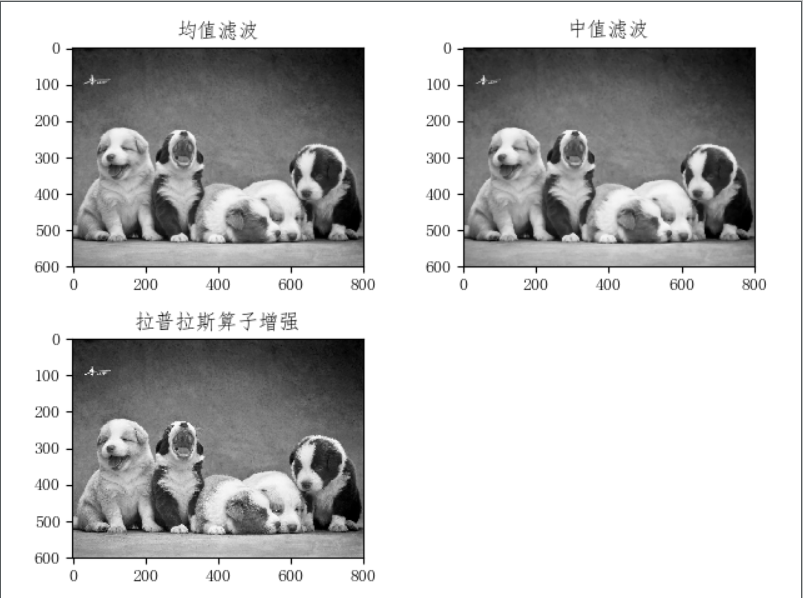参考链接


展开全文计算机视觉 python
• 图像预处理源代码 图像预处理源代码 图像预处理源代码
• 基于Matlab的火灾图像预处理-基于Matlab的火灾图像预处理.pdf 摘 要:本文研究了在Matlab 环境下如何对图像,特别是火灾图像进行预处理。预处理的过程分为两个步骤,包括火灾图像的增强和滤波。用一些Matlab 的处理...matlab
• ## 指纹图像预处理

千次阅读 多人点赞 2020-10-16 16:54:31
指纹图像预处理是指纹识别的前提,它的好坏直接影响到指纹识别的成败，但由于指纹图像降质带来的困难,并根据指纹图像的特征提出了合理的假设，再根据假设提出了增强指纹图像对比度的算法、提取指纹有效区域的算法、...
    指纹图像预处理是指纹识别的前提,它的好坏直接影响到指纹识别的成败，但由于指纹图像降质带来的困难,并根据指纹图像的特征提出了合理的假设，再根据假设提出了增强指纹图像对比度的算法、提取指纹有效区域的算法、根据方向信息分割图像的算法以及去除图像中气泡噪声的算法，这些算法处理效果好，能有效地解决指纹图像的预处理问题。

用Matlab实现这种方法，既能分步对指纹图像预处理算法进行仿真测试，又可以很直观地看到图像预处理算法的效果。实验证明，用Matlab实现的处理结果比较理想，满足识别的应用性。本文介绍用matlab实现了指纹图像的对比度增强、有效区域的选取、指纹图像的二值化、指纹的特征值提取等。并选取较好的处理步骤和算法参数解决指纹图像预处理的问题。

指纹是人类手指末端指腹上由凹凸的皮肤所形成的纹路。指纹能使手在接触物件时增加摩擦力，从而更容易发力及抓紧物件。是人类进化过程式中自然形成的。目前尚未发现有不同的人拥有相同的指纹，所以每个人的指纹也是独一无二。由于指纹是每个人独有的标记，近几百年来，罪犯在犯案现场留下的指纹，均成为警方追捕疑犯的重要线索，使得指纹识别技术得到了飞快的发展。现今鉴别指纹方法已经电脑化，使鉴别程序更快更准。

指纹识别技术源于19世纪初，科学家依靠指纹纹脊式样的唯一性和式样终生不改变的特性，把某个人同他的指纹对应起来，通过采集他的指纹并与预先保存的指纹进行比较来验证其真实身份。随着现代科技的不断进步与广泛应用，可靠高效的个人身份识别变得越来越需要，每个人的指纹具有惟一性，终身不变，难以伪造，因此指纹识别是替代传统身份识别手段的最安全、最可靠、最方便的方法。指纹图像本身的信息量和数据量是很大的因此直接基于指纹图象的匹配识别是不可取的，而要采用专门高教的指纹识别与处理方法。

指纹识别的一般过程是指纹图象预处理、指纹特征提取和特征匹配。但由于采集设备噪声干扰、指纹采集时手指皮肤的干燥程度、汗渍、污渍等原因使待分析的指纹图像噪声较多并对细节点有较强干扰，影响指纹的特征提取。指纹图像是通过将模拟信号采样量化后，以矩阵形式存入计算机，图像平滑处理指纹图像生成方向数组后，为了消除较强烈的局部噪声干扰，需要对生成的方向数组图像进行预处理。预处理是指纹识别的前提，也是整个工作的基础，因此指纹图象预处理工作的好坏直接关系到指纹特征提取的可行性和准确性。

指纹是手指末端正面皮肤上凹凸不平产生的纹路，这些纹路就是通常所说的脊和谷。指纹虽小，但它蕴涵了大量信息。其中，包括纹型在内的全局特征，为指纹的分类提供了基础；同样，指纹还有许多局部特征(根据美国国家标准局规定，包括脊末梢、分岔点、复合特征和未定义四种)，称为细节点(Minutia)。不同人的指纹的细节点是唯一的、稳定不变的，这为指纹识别提供了可能。目前，最常用的方法是用FBI提出的指纹细节点模型来做细节匹配。而最常用的细节特征有脊末梢和分支点两种。

基于点模式匹配的自动指纹识别系统(AFIS)的基本流程一般由图像采集、图像预处理、细节点提取和指纹匹配几部分组成。

首先，指纹要通过指纹采集设备(常见的有光学取像设备、超声波扫描取像设备、晶体传感器，现在广泛使用的是晶体传感器)转化为计算机内的数字图像(一般为灰度图)。由于采集过程中难免因手指或仪器的原因而使图像存在较多的噪声，所以为了使图像更清晰以便于后续特征提取，必须对采集到的图像进行增强和滤波，并进一步二值化、细化。

之后，在细化后的点线图上提取特征值，删除伪特征值，最终得到用于匹配的细节点。采集到的图像细节点与模板中的细节点进行比对，最终完成指纹匹配。各个环节环环相扣，对整个系统都起着十分重要的作用。本文着重研究了图像预处理和细节特征提取这两个关键部分。

而在指纹采集过程中，不可避免的会引入各种噪声，如图像中的叉连、断点等，这些噪声对指纹特征信息的提取造成一定的影响，甚至会产生许多伪特征点。因此在提取指纹特征之前，需要对指纹图像进行滤波处理，以去除无用信息，增强有用信息。在得到增强的灰度图后，需要将其进一步二值化，便于后续过程的处理。指纹图像预处理是去除指纹图像中的噪声，使指纹图像清晰、边缘明显，以便于提高提取和存储特征点的准确率。包括指纹区域检测、图像质量判断、方向图和频率估计、图像增强、指纹图像二值化和细化等。

指纹图像获取是通过专门的指纹采集仪可以采集活体指纹图像。目前，指纹采集仪主要有活体光学式、电容式和压感式。对于分辨率和采集面积等技术指标，公安行业已经形成了国际和国内标准，但其他还缺少统一标准。根据采集指纹面积大体可以分为滚动捺印指纹和平面捺印指纹，公安行业普遍采用滚动捺印指纹。另外，也可以通过扫描仪、数字相机等获取指纹图像。

指纹图像只有脊和谷之分， 因此完全可以由二值图象来描述，也就是指纹图像的二值化。目前指纹的二值化不外乎两种方法， 一种是固定门限法， 另一种是动态门限法。固定门限法是对整幅图象用一个灰度门限值， 它对输入图象要求高， 要求整幅图象灰度分布均匀。因此我们把均衡增强后的图象作为它的输入图象。

动态门限法是根据不同区域取不同门限值，一般采用平均域值法。它对输入图象照射要求不高。因此我们把方向性滤波后图象作为输入图象。

指纹图像的细化是找出指纹纹线的轴心线来代替纹线的过程。目前采用的细化方法就是迭代一一轮廓剥离法。每次垒图象扫描迭代一次，就剥掉边界象素中不影响连通性的象素，直至纹线宽度为1个象素为止。这样一来，如果被细化的纹线宽度越厚，迭代次数就越多，细化时间就越长，这是我们不希望的。

指纹形态特征包括中心（上、下）和三角点（左、右）等，指纹的细节特征点主要包括纹线的起点、终点、结合点和分叉点。将这些点进行自动选择从而完成指纹形态和细节特征提取的工作。

指纹比对是将可以根据指纹的纹形进行粗匹配，进而利用指纹形态和细节特征进行精确匹配，给出两枚指纹的相似性得分。根据应用的不同，对指纹的相似性得分进行排序或给出是否为同一指纹的判决结果。

1.5 指纹图像预处理过程及一般算法

在指纹图象处理的流程中，预处理是第一个处理环节．它对原始灰度图像进行平滑、锐化、增强、二值化等处理，从而使细化、特征抽取等操作能够有效进行。

在常见的图象处理技术中，通常按处理目的把预处理过程分为平滑、增强、二值化等步骤。每一步骤都有一些常用算法，如用于平滑的均值滤波法、中值滤波法、迭代加权法等，用于增强的规格化法、自适应算法、拉普拉斯法、Wdlis滤波、Lee滤波等。

经过很长时间的深入研究和反复实践，发现这些常用的算法应用在指纹图象处理中有下列的问题：

(1)这些算法对于指纹图象处理的效果并不理想，尽管从视觉上有一定改善，但对于后续的细化和特征抽取处理效果来看，不能有效地提高特征的准确率。

(2)不能较好地处理指纹的背景部分，严重影响特征抽取和识别。

(3)不能根据指纹图象的质量差别进行特殊处理，通常获得的指纹图象，会有部分区域质量较差，无法抽取特征，在这些算法中，无法找到一个判别标准。

对此，则需要我们对算法原理的进一步了解及改进。

方向图算法正是基于以上特点在80年代初期，就已经开始有把方向图引入到指纹图的一些成功的尝试。这时候所使用的方向图是从二值图中直接提取，得到的处理效果并不完全令人满意。

从1987年开始，B M．Mehtre等人成功地得到了在灰度图上直接获取方向图的有效算法，并陆续提出了一系列的预处理方法来处理指纹灰度图。使用这些算法使指纹图象的处理效果达到了一个新的水平，从而使基于方向图的算法成为指纹图象处理方法研究中的一个热点 在以后的研究中，出现了很多改进和发展，如Kallen Karu等1996 年提出的把方向图用于纹型分类。Linghong等1998年提出的基于方向图的纹线增强等都取得了较好的效果。这使得方向图成为指纹图象处理技术的关键技术之一。

目前的基于方向图的算法中，从灰度图中获取方向图的原理都大体相同，其基本原理是：从图象的灰度矩阵C(I，J)中计算在各个方向上的某个统计量如灰度差或灰度平均等，根据这些统计量在各个方向上的差异，确定在一个小临域内纹线的主方向。针对每象素得到的方向则形成点方向图。为了保持点方向的有效性使用方便，对点方向在一小块内聚类则得到块方向图。

1.6特征拾取、验证和辨识

一个高质量的图像被拾取后，需要许多步骤将它的特征转换到一个复合的模板中，这个过程被称为特征拾取过程，它是手指扫描技术的核心。当一个高质量的图像被拾取后，它必须被转换成一个有用的格式。如果图像是灰度图像，相对较浅的部分会被删除，而相对较深的部分被变成了黑色。脊的像素有5~8个被缩细到一个像素，这样就能精确定位脊断点和分岔了。微小细节的图像便来自于这个经过处理的图像。在这一点上，即便是十分精细的图像也存在着变形细节和错误细节，这些变形和错误细节都要被滤除。

除细节的定位和夹角方法的应用以外，也可通过细节的类型和质量来划分细节。这种方法的好处在于检索的速度有了较大的提高，一个显著的、特定的细节，它的惟一性更容易使匹配成功。还有一些生产商采用的方法是模式匹配方法，即通过推断一组特定脊的数据来处理指纹图像。

就应用方法而言，指纹识别技术可分为验证和辨识。

验证就是通过把一个现场采集到的指纹与一个已经登记的指纹进行一对一的比对来确定身份的过程。指纹以一定的压缩格式存储，并与其姓名或其标识（ID，PIN）联系起来。随后在对比现场，先验证其标识，然后利用系统的指纹与现场采集的指纹比对来证明其标识是合法的。验证其实回答了这样一个问题："他是他自称的这个人吗？"这是应用系统中使用得较多的方法。

辨识则是把现场采集到的指纹同指纹数据库中的指纹逐一对比，从中找出与现场指纹相匹配的指纹。这也叫"一对多匹配"。辨识其实是回答了这样一个问题："他是谁？"

指纹是人体独一无二的特征，其复杂度足以提供用于鉴别的特征。随着相关支持技术的逐步成熟，指纹识别技术经过多年的发展已成为目前最方便、可靠、非侵害和价格便宜的生物识别技术解决方案，对于广大市场的应用有着很大的发展潜力。

1.7 指纹识别的主要应用

指纹识别键盘现在的计算机应用中，包括许多非常机密的文件保护，大都使用“用户ID+密码”的方法来进行用户的身份认证和访问控制。但是，如果一旦密码忘记，或被别人窃取，计算机系统以及文件的安全问题就受到了威胁。

随着科技的进步，指纹识别技术已经开始慢慢进入计算机世界中。目前许多公司和研究机构都在指纹识别技术领域取得了很大突破性进展，推出许多指纹识别与传统IT技术完美结合的应用产品，这些产品已经被越来越多的用户所认可。指纹识别技术多用于对安全性要求比较高的商务领域，而在商务移动办公领域颇具建树的富士通、三星及IBM等国际知名品牌都拥有技术与应用较为成熟的指纹识别系统，下面就对指纹识别系统在笔记本电脑中的应用进行简单介绍。

众所周知，在两年前就有部分品牌的笔记本采用指纹识别技术用于用户登录时的身份鉴定第一代光学式指纹读取器，但是，当时推出的指纹系统属于光学识别系统，按照现在的说法，应该属于第一代指纹识别技术。光学指纹识别系统由于光不能穿透皮肤表层（死性皮肤层），所以只能够扫描手指皮肤的表面，或者扫描到死性皮肤层，但不能深入真皮层。

在这种情况下，手指表面的干净程度，直接影响到识别的效果。如果，用户手指上粘了较多的灰尘，可能就会出现识别出错的情况。并且，如果人们按照手指，做一个指纹手模，也可能通过识别系统，对于用户而言，使用起来不是很安全和稳定。

因此出现了第二代电容式传感器，电容传感器技术是采用了交替命令的并排列和传感器电板，交替板的第二代电容式传感器形式是两个电容板，以及指纹的山谷和山脊成为板之间的电介质。两者之间的恒量电介质的传感器检测变化来生成指纹图像。但是由于传感器表面是使用硅材料容易损坏，导致使用寿命降低，还有它是通过指纹的山谷和山脊之间的凹凸来形成指纹图像的，所以对脏手指、湿手指等困难手指识别率低。

发展到今天，出现第三代生物射频指纹识别技术，射频传感器技术是通过传感器本身发射出微量射频信号，穿透手指的表皮层去控测里层的纹路，来获得最佳的指纹图像。因此对干手指，汉手指等困难手指通过可高达99%，防伪指纹能力强，指纹敏感器的识别原理只对人的真皮皮肤有反应，从根本上杜绝了人造指纹的问题，宽温区：适合特别寒冷或特别酷热的地区。因为射频传感器产生高质量的图像，因此射频技术是最可靠，最有力有解决方案。除此之外，高质量图像还允许减小传感器，无需牺牲认证的可靠性，从而降低成本并使得射频传感器思想的应用到可移动和大小不受拘束的任何领域中。

指纹识别技术还可以通过几种方法应用到许多方面。可以想象如果计算机上的所有系统和应用程序都可以使用指纹验证的话，人们使用计算机就会非常方便和安全，用户不再讨厌必要的安全性检查，而IT开发商的售后服务工作也会减轻许多。IBM公司已经开发成功并广泛应用的Global Sign On软件通过定义唯一的口令，或者使用指纹，就可以在公司整个网络上畅行无阻。 把指纹识别技术同IC卡结合起来，是目前最有前景的一个方向之一。该技术把卡的主人的指纹（加密后）存储在IC卡上，并在IC卡的读卡机上加装指纹识别系统，当读卡机阅读卡上的信息时，一并读入持卡者的指纹，通过比对卡上的指纹与持卡者的指纹就可以确认持卡者的是否卡的真正主人，从而进行下一步的交易。在更加严格的场合，还可以进一步同后端主机系统数据库上的指纹作比较。指纹IC卡可以广泛地运用于许多行业中，例如取代现行的ATM卡、制造防伪证件（签证或护照、公费医疗卡、会员卡、借书卡等）。目前ATM提款机加装指纹识别功能在美国已经开始使用。持卡人可以取消密码 (避免老人和孩子记忆密码的困难)或者仍旧保留密码，在操作上按指纹与密码的时间差不多。 　　

近年来，自动发送信息的互联网络，带给人们的方便与利益，正在快速增长之中，但也因此产生了很多的问题，尤其在信息安全方面。无论是团体或者个人的信息，都害怕在四通八达的网络上传送而发生有损权益的事情。由于指纹特征数据可以通过电子邮件或其他传输方法在计算机网络上进行传输和验证，通过指纹识别技术，限定只有指定的人才能访问相关信息，可以极大地提高网上信息的安全性，这样，包括网上银行、网上贸易、电子商务的一系列网络商业行为，就有了安全性保障。在SFNB(Security First Network Bank安全第一网络银行)，就是通过互联网络来进行资金划算的，他们目前正在实施以指纹识别技术为基础的保障安全性的项目，以增强交易的安全性。 　　

在医院里，指纹识别技术可以验证病人身份，例如输血管理。指纹识别技术也有助于证实寻求公共救援、医疗及其他政府福利或者保险金的人的身份确认。在这些应用中，指纹识别系统将会取代或者补充许多大量使用照片和ID的系统。 　　总之，随着许多指纹识别产品已经开发和生产，指纹识别技术的应用已经开始进入民用市场，并且发展迅猛，相信这一技术的普及应用已经指日可待。

如果在开始将D的值进行改变，将其变小为5时，可以得到更多的特征值，因为D的改变，将导致特征值点的取值改变，其得到的图像如下图3.10所示：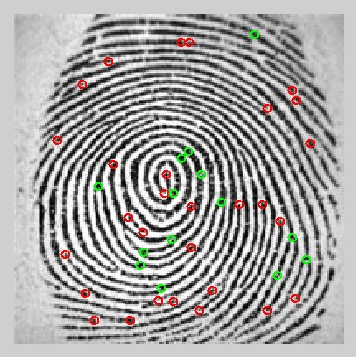图3.10与图3.8相比，图3.10中绿色的交叉点跟红色的起始点与终结点都多了一些，而这些多是伪特征点，如3.11所示：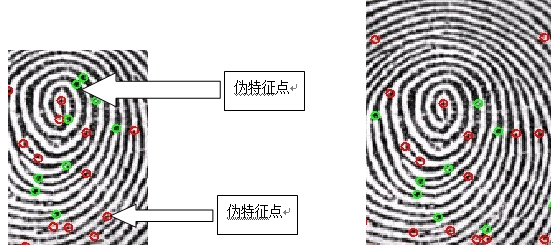MATLAB实验主程序：

clear all,close all,clc

% The general shape of the fingerprint is generally used to pre-process the

% images, and reduce the search in large databases. This uses the general

% directions of the lines of the fingerprint, and the presence of the core

% and the delta. Several categories have been defined in the Henry system:

% whorl, right loop, left loop, arch, and tented arch. Ö¸ÎÆ·ÖÀàÓÐÖúÓÚ¼õÉÙËÑË÷ËÙ¶È

%

% Most algorithms are using minutiae, the specific points like ridges

% are stored in the signature for further comparison.

imshow(I)

set(gcf,'position',[1 1 600 600]);

%% EnhancementÖ¸ÎÆÔöÇ¿

% A critical step in automatic fingerprint matching is to automatically and

% reliably extract minutiae £¨Ï¸½ÚÌáÈ¡£©from the input fingerprint images. However, the

% performance£¨ÐÔÄÜ£© of a minutiae extraction algorithm relies heavily on the

% quality £¨ÓëÖ¸ÎÆÖÊÁ¿ÓÐ¹Ø£©of the input fingerprint images. In order to ensure that the

% performance of an automatic fingerprint identification/verification system

% would be robust with respect to the quality of the fingerprint images, it

% should be essential to incorporate a fingerprint enhancement algorithm in the

%

% In our case, the quality of the image is really good, and we wwon't need

%% Binarize(¶þÖµ»¯)

% We binarize the image. After the operation, ridges in the fingerprint are

% highlighted with black color while furrow are white.¶þÖµ»¯ºó¼¹ÎªºÚÉ«,ÖåñÞÎª°×É«

J=I(:,:,1)>160;

imshow(J)

set(gcf,'position',[1 1 600 600]);

%% Thining(Ï¸»¯)

% Ridge thining is to eliminate the redundant pixels of ridges till the

% ridges are just one pixel wide.

K=bwmorph(~J,'thin','inf');

imshow(~K)

set(gcf,'position',[1 1 600 600]);

%% Minutiae(Ï¸½ÚÌáÈ¡Ä£°æ)

% We filter the thinned ridge map by the filter "minutie". "minutie"

% compute the number of one-value of each 3x3 window:

% * if the central is 1 and has only 1 one-value neighbor, then the central

% pixel is a termination(ÅÐ¶Ï¶ËµãµÄ·½·¨).

% * if the central is 1 and has 3 one-value neighbor, then the central

% pixel is a bifurcation(ÅÐ¶Ï·Ö²æµã·½·¨).

% * if the central is 1 and has 2 one-value neighbor, then the central

% pixel is a usual pixel.

fun=@minutie;

L = nlfilter(K,[3 3],fun);

%% Termination

LTerm=(L==1);%ÖÐÐÄµãÅÔ±ßÓÐÒ»¸öµã

imshow(LTerm)

LTermLab=bwlabel(LTerm);

propTerm=regionprops(LTermLab,'Centroid');

CentroidTerm=round(cat(1,propTerm(:).Centroid));

imshow(~K)

set(gcf,'position',[1 1 600 600]);

hold on

plot(CentroidTerm(:,1),CentroidTerm(:,2),'ro')

%% Bifurcation

LBif=(L==3);

LBifLab=bwlabel(LBif);

propBif=regionprops(LBifLab,'Centroid','Image');%Measure properties of image regions

CentroidBif=round(cat(1,propBif(:).Centroid));%Round to nearest integer

plot(CentroidBif(:,1),CentroidBif(:,2),'go')

%% Remarks

% We have a lot of spurious minutae.

% We are going to process them.

% process 1: if the distance between a termination and a biffurcation is

% smaller than D, we remove this minutiae

% process 2: if the distance between two biffurcations is

% smaller than D, we remove this minutia

% process 3: if the distance between two terminations is

% smaller than D, we remove this minutia

D=6;

%% Process 1

Distance=DistEuclidian(CentroidBif,CentroidTerm);

SpuriousMinutae=Distance<D;

[i,j]=find(SpuriousMinutae);

CentroidBif(i,:)=[];

CentroidTerm(j,:)=[];

%% Process 2

Distance=DistEuclidian(CentroidBif);

SpuriousMinutae=Distance<D;

[i,j]=find(SpuriousMinutae);

CentroidBif(i,:)=[];

%% Process 3

Distance=DistEuclidian(CentroidTerm);

SpuriousMinutae=Distance<D;

[i,j]=find(SpuriousMinutae);

CentroidTerm(i,:)=[];

%%

hold off

imshow(~K)

hold on

plot(CentroidTerm(:,1),CentroidTerm(:,2),'ro')

plot(CentroidBif(:,1),CentroidBif(:,2),'go')

hold off

%% ROI

% We have to determine a ROI. For that, we consider the binary image, and

% we aply an closing on this image and an erosion.

% With the GUI, I allow the use of ROI tools of MATLAB, to define manually

% the ROI.

Kopen=imclose(K,strel('square',7));

KopenClean= imfill(Kopen,'holes');

KopenClean=bwareaopen(KopenClean,5);

imshow(KopenClean)

KopenClean([1 end],:)=0;

KopenClean(:,[1 end])=0;

ROI=imerode(KopenClean,strel('disk',10));

imshow(ROI)

%%

imshow(I)

hold on

imshow(ROI)

alpha(0.5)

hold on

plot(CentroidTerm(:,1),CentroidTerm(:,2),'ro')

plot(CentroidBif(:,1),CentroidBif(:,2),'go')

hold off

%% Suppress extrema minutiae

% Once we defined the ROI, we can suppress minutiae external to this ROI.

[m,n]=size(I(:,:,1));

indTerm=sub2ind([m,n],CentroidTerm(:,1),CentroidTerm(:,2));

Z=zeros(m,n);

Z(indTerm)=1;

ZTerm=Z.*ROI';

[CentroidTermX,CentroidTermY]=find(ZTerm);

indBif=sub2ind([m,n],CentroidBif(:,1),CentroidBif(:,2));

Z=zeros(m,n);

Z(indBif)=1;

ZBif=Z.*ROI';

[CentroidBifX,CentroidBifY]=find(ZBif);

imshow(I)

hold on

plot(CentroidTermX,CentroidTermY,'ro','linewidth',2)

plot(CentroidBifX,CentroidBifY,'go','linewidth',2)

%% Orientation

% Once we determined the differents minutiae, we have to find the

% orientation of each one

Table=[3*pi/4 2*pi/3 pi/2 pi/3 pi/4

5*pi/6 0 0 0 pi/6

pi 0 0 0 0

-5*pi/6 0 0 0 -pi/6

-3*pi/4 -2*pi/3 -pi/2 -pi/3 -pi/4];

%% Termination Orientation

% We have to find the orientation of the termination.

% For finding that, we analyze the position of the pixel on the boundary of

% a 5 x 5 bounding box of the termination. We compare this position to the

% Table variable. The Table variable gives the angle in radian.

for ind=1:length(CentroidTermX)

Klocal=K(CentroidTermY(ind)-2:CentroidTermY(ind)+2,CentroidTermX(ind)-2:CentroidTermX(ind)+2);

Klocal(2:end-1,2:end-1)=0;

[i,j]=find(Klocal);

OrientationTerm(ind,1)=Table(i,j);

end

dxTerm=sin(OrientationTerm)*5;

dyTerm=cos(OrientationTerm)*5;

figure

imshow(K)

set(gcf,'position',[1 1 600 600]);

hold on

plot(CentroidTermX,CentroidTermY,'ro','linewidth',2)

plot([CentroidTermX CentroidTermX+dyTerm]',...

[CentroidTermY CentroidTermY-dxTerm]','r','linewidth',2)

%% Bifurcation Orientation

%  For each bifurcation, we have three lines. So we operate the same

%  process than in termination case three times.

for ind=1:length(CentroidBifX)

Klocal=K(CentroidBifY(ind)-2:CentroidBifY(ind)+2,CentroidBifX(ind)-2:CentroidBifX(ind)+2);

Klocal(2:end-1,2:end-1)=0;

[i,j]=find(Klocal);

if length(i)~=3

CentroidBifY(ind)=NaN;

CentroidBifX(ind)=NaN;

OrientationBif(ind)=NaN;

else

for k=1:3

OrientationBif(ind,k)=Table(i(k),j(k));

dxBif(ind,k)=sin(OrientationBif(ind,k))*5;

dyBif(ind,k)=cos(OrientationBif(ind,k))*5;

end

end

end

plot(CentroidBifX,CentroidBifY,'go','linewidth',2)

OrientationLinesX=[CentroidBifX CentroidBifX+dyBif(:,1);CentroidBifX CentroidBifX+dyBif(:,2);CentroidBifX CentroidBifX+dyBif(:,3)]';

OrientationLinesY=[CentroidBifY CentroidBifY-dxBif(:,1);CentroidBifY CentroidBifY-dxBif(:,2);CentroidBifY CentroidBifY-dxBif(:,3)]';

plot(OrientationLinesX,OrientationLinesY,'g','linewidth',2)

附录2

变量D的定义程序：

function D=DistEuclidian(dataset1,dataset2)

h = waitbar(0,'Distance Computation');

switch nargin

case 1

[m1,n1]=size(dataset1);

m2=m1;

D=zeros(m1,m2);

for i=1:m1

waitbar(i/m1)

for j=1:m2

if i==j

D(i,j)=NaN;

else

D(i,j)=sqrt((dataset1(i,1)-dataset1(j,1))^2+(dataset1(i,2)-dataset1(j,2))^2);

end

end

end

case 2

[m1,n1]=size(dataset1);

[m2,n2]=size(dataset2);

D=zeros(m1,m2);

for i=1:m1

waitbar(i/m1)

for j=1:m2

D(i,j)=sqrt((dataset1(i,1)-dataset2(j,1))^2+(dataset1(i,2)-dataset2(j,2))^2);

end

end

otherwise

error('only one or two input arguments')

end

close(h)

附录3

指纹图像二值化，细化程序：

subplot(421);

imshow(I);

set(gcf,'position',[1 1 600 600]);

J=I(:,:,1)>160;

subplot(322);imshow(J)

set(gcf,'position',[1 1 600 600])

K=bwmorph(~J,'thin','inf');

subplot(323);imshow(~K)

set(gcf,'position',[1 1 600 600]);

fun=@minutie;

L = nlfilter(K,[3 3],fun);

%LTerm=(L==1);

%subplot(324);

%imshow(LTerm);

LTermLab=bwlabel(J);

subplot(324);

imshow(LTermLab)

展开全文...